# Circle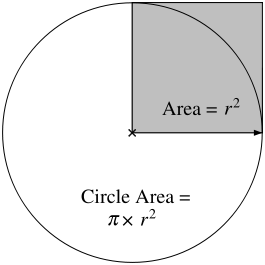Area of the circle =× area of the shaded square

A circle is the set of all the points in a given plane that are the same distance, called the radius, r, from a given point called the center.

A circle may also be defined algebraically, as the set of solutions to an equation of the form:,

whereis the center of the circle. It can also be described parametrically in terms of a parameteras: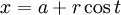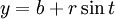The distance around the circle, or circumference, is given by: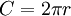The area inside the circle is calculated using the formula:This can be easily derived from the area of an ellipse. For an ellipse with major and minor axisandrespectively, the area is. Setting the major an minor axis equal to each other give the formula for a circle.

A circle is a conic section, the intersection of a plane with a cone such that the plane is perpendicular to the axis of the cone.

Circles can readily be constructed by using a fixed distance between a pencil point and the center. A compass is a tool for doing this easily.

A circle with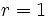is called the unit circle, and is used extensively in trigonometry.